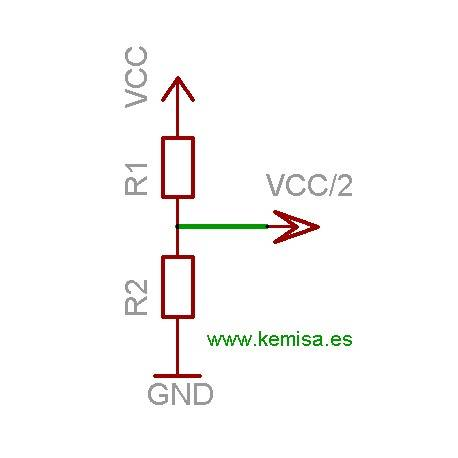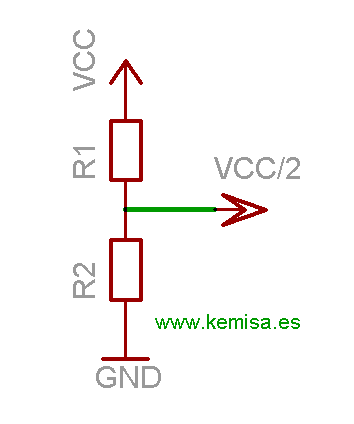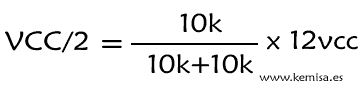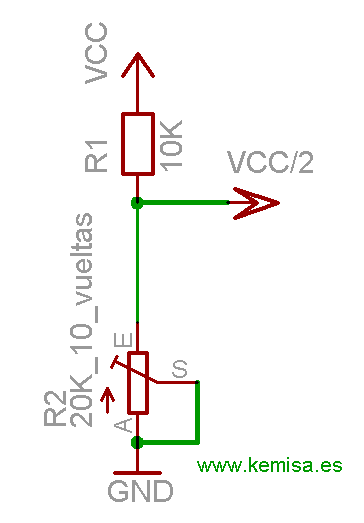## Electronics tutorials

Viewed productsView larger

# How to make a voltage divider

#### The resistive divider consists of two resistors, connected like in the following image scheme.#### Joining a voltage to the point VCC, we obtain the voltage reduction at the point VCC/2. To calculate the voltage decrease at the point VCC/2, we apply this formula:#### For example, we have a 12 volt power at the VCC entrance and we wish to reduce it to 6 volts at VCC/2. We shall put that R1=10k and R2=10k. According to the formula:#### This way we vary the trimmer until we obtain exactly 6 volts at the exit VCC/2.